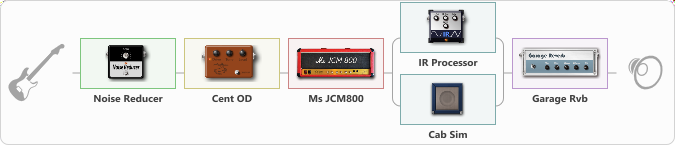# rock

Discussion in 'ToneLib-GFX presets' started by carlinos3, Feb 17, 2020.

1. rock

Preset name: Metal Rhythm

Effects chain:Effect: "Noise Reducer" (Dynamics / Filter), active - "yes"
{
"Sens" = 81
"Mode" = Soft
}

Effect: "Cent OD" (Overdrive / Distortion), active - "yes"
{
"Drive" = 52
"Tone" = 70
"Level" = 74
}

Effect: "Ms JCM800" (Amp simulators), active - "yes"
{
"Gain" = 100
"Bass" = 82
"Middle" = 23
"Treble" = 39
"Presence" = 65
"Master" = 78
"Level (dB)" = 2
}

Effect: "Splitter" (Dynamics / Filter), active - "yes"
{
"A-Bypass" = Off
"A-Pan" = -42
"A-Level" = 55
"B-Bypass" = Off
"B-Pan" = 50
"B-Level" = 55

Left branch:
{

Effect: "IR Processor" (Cabinets), active - "yes"
{
"IR" = carlos-7
"Low Cut (Hz)" = 0
"Hi Cut (kHz)" = 20.0
"Mix" = 100
"Level (dB)" = 10
}
}
Right branch:
{

Effect: "Cab Sim" (Cabinets), active - "yes"
{
"Model" = 4x12" 1960 T75
"Level (dB)" = 7
}
}
}

Effect: "Garage Rvb" (Reverb), active - "yes"
{
"Time" = 5.2
"PreDelay" = 46
"LoDamp" = 0
"HiDamp" = 30
"Mix" = 54
}

Note: You will need to download and install the ToneLib-GFX software to use the preset.

File size:
3.6 KB
Views:
4,580